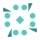Home
IT Knowledge
Inspiration
Languages
EN

# Java - initialize HashSet at construction

0 points
Created by:kaya31
336

In this article, we would like to show you how to initialize HashSet at the construction in Java.

Quick solution:

``Set<String> letters = new HashSet<>(Arrays.asList("A", "B", "C"));``

## 1. Initialize HashSet using `Arrays.asList()`

In this example, to initialize `letters` HashSet at the construction time, we use `Arrays.asList()` method in the following way:

``````import java.util.*;

public class Example {

public static void main(String[] args) {
Set<String> letters = new HashSet<>(Arrays.asList("A", "B", "C"));

System.out.println(letters);
}
}``````

Output:

``[A, B, C]``

Note:

This approache is inefficient since it needs to create an array, convert it to a list, and use it to create a HashSet.

## 2. Initialize HashSet using anonymous class

In this example, we use double curly braces to initialize `letters` HashSet on its creation.

``````import java.util.*;

public class Example {

public static void main(String[] args) {
Set<String> letters = new HashSet<>() {{
}};

System.out.println(letters);
}
}``````

Output:

``[A, B, C]``

Note:

This approache is inefficient since it needs to create anonymous class each time it's called